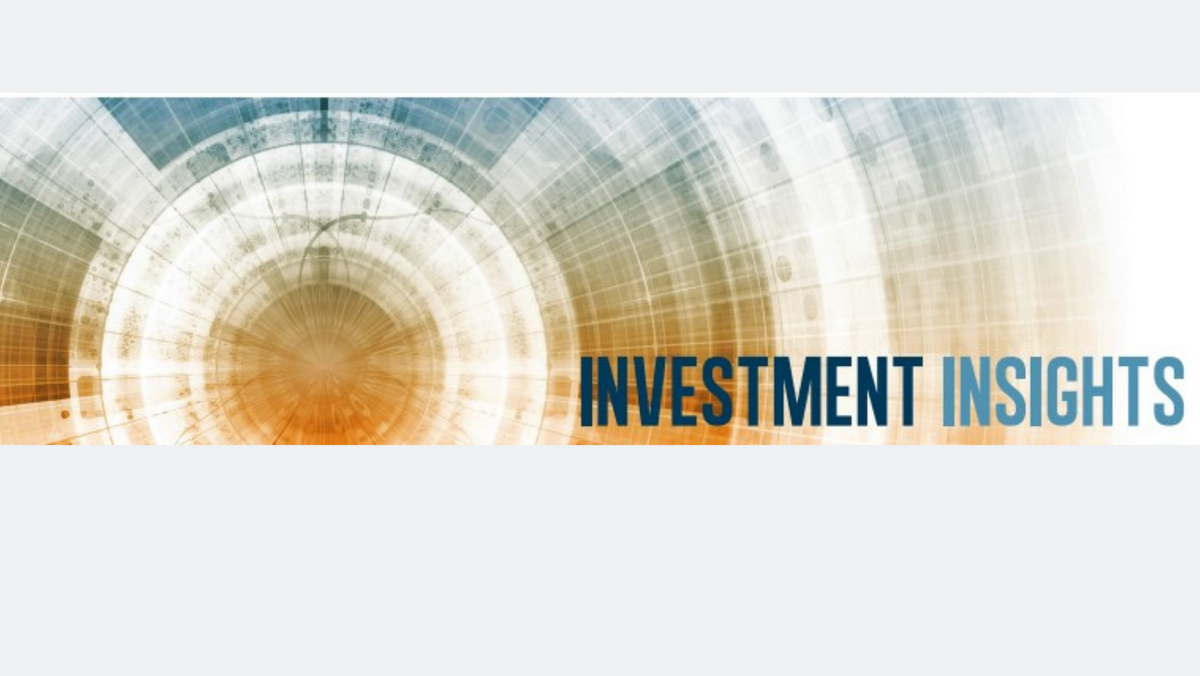# Investment Insights - June 2022

June 15, 2022

## Rising Rates & Fixed Income Investing

As the economy picks up steam with inflation well above its target, the Fed is on the path toward raising interest rates to slow a potential overheating of the economy. With interest rates normalizing to a higher level, many investors may worry about the interest rate risk of their bond investments. This issue examines the interest rate risk of bond investments.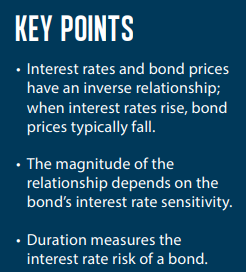### The Inverse Relationship

Interest rates and bond prices have an inverse relationship. When the interest rate (or the cost of borrowing money) increases, the bond price typically declines. This relationship is generally not intuitive for many people. The following is an example to help us understand this inverse relationship from a bondholder's perspective. Imagine you own a bond (Bond A), and interest rates rise. Given the higher interest rates, a new bond (Bond B) issued in the market will have higher yields as compared to Bond A. Therefore, Bond A is now worth less than Bond B. When the interest rate increases, the bond price goes down. Assume instead that the interest rates decrease. In this case, the new bonds in the market will have lower yields, making the older bonds more valuable. This results in higher bond prices.

Using the same example with hypothetical numbers can help further clarify this relationship. Imagine you own a bond (Bond A) that has a 4% coupon or interest rate. Later, the prevailing interest rates increase such that a newly issued similar bond (Bond B) now has an interest rate of 4.5%. Since people would rather own Bond B (as it pays more), Bond A is less valuable than Bond B. Thus, the price of the bond you own decreases. Assume Bond A is a \$1,000 bond with a 4% coupon that pays \$40 per year (\$1000 times 4%). If interest rates increase such that a similar bond now pays 4.5% (to attract buyers), the price of Bond A must decline by \$111 to make the yield equal between Bond A and Bond B. After the price adjusts from \$1,000 to \$888 by subtracting \$111, the yield of Bond A becomes 4.5% (\$40/\$888).

It is helpful to think of this inverse relationship using a seesaw with bond  price on one end and interest rates on the other. When one side increases, the other side typically declines, creating an inverse relationship between bond price and interest rate.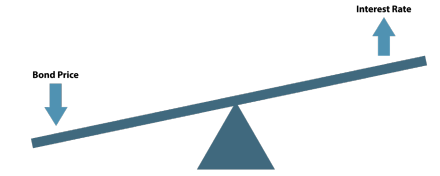### MEASURING INTEREST RATE RISK

While we know the relationship between bond prices and interest rates, we still need to understand the magnitude of this relationship. Not all bond prices are expected to have the same inverse relationship with interest rates. Some bonds are more sensitive to interest rate changes than others. The measure of how sensitive a bond is to interest rates is called duration. More precisely, it measures the percentage change in bond price for a one percentage point change in interest rates. Bonds with higher duration are more sensitive to interest rate change and have higher interest rate risk. Similarly, bonds with lower duration are less sensitive to interest rate changes and have lower interest rate risk.

The main driver of bond duration is the maturity of the bond. All else equal, bonds with longer maturity typically have higher duration than bonds with lower maturity. The graph below shows the duration of various U.S. Treasuries. The bonds that mature sooner, like the 2-year T-Note, have a smaller duration, whereas 30-year T-Bonds have a larger duration.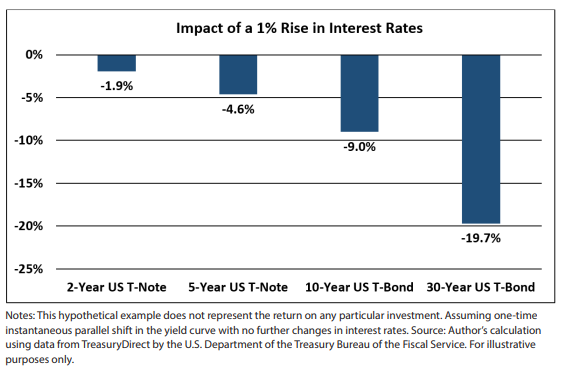### MANAGING INTEREST RATE RISK

Rising interest rates may concern many bond investors. As we discussed above, rising interest rates can result in falling bond prices. This can have a negative impact on performance returns for bond investors. Consequently, interest rate risk should be an important consideration for bond investors.

Using the concept of duration discussed above, the chart below shows the estimated drop in various types of bond prices, assuming interest rates rise by 1%. In this hypothetical example, the yield is assumed at 2.45%, 2.72%, and 2.85% for the 2,5, and 10-year treasuries. With a 1% rise in interest rates, you can see that the bond with the highest duration falls the most since it is the most sensitive to interest rate change.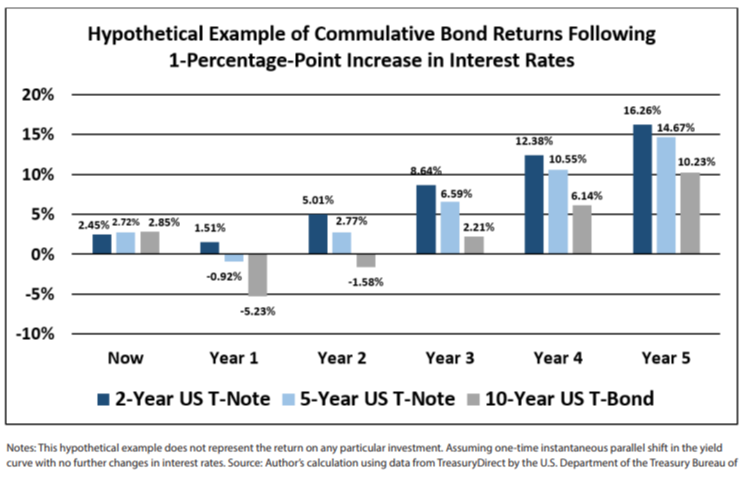An interesting thing to note in the graph above is that while returns initially declined on a cumulative basis, the returns became positive in the proceeding years. After the interest rate increases, bond investors enjoy higher returns on their reinvestments. For long-term investors, rising interest rates can be good for bond returns as they provide higher returns on their investment over time.

It is crucial to manage your bond investments’ interest rate risk carefully. Taking too much interest rate risk coupled with a large duration and time horizon mismatch can be potentially disastrous. For example, the following graph extends the one above and shows the impact of a 1% interest rate increase on 30-year treasuries. Given the longer duration of the 30-year bond, a one percent increase in interest rate is expected to result in prices declining more than 15%. This decline is large enough that even after five years, the cumulative bond returns are not positive in this hypothetical example.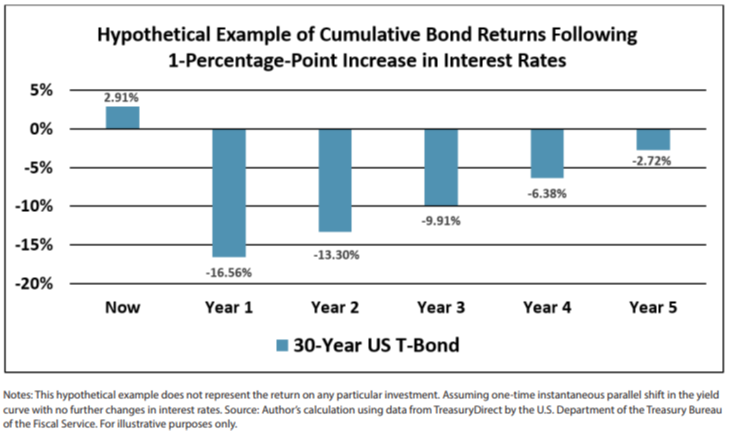### INTEREST RATE RISK AND YOUR INVESTMENTS

The rising interest rate environment may produce higher than normal chances of bond prices declining. As such, it is important to manage the interest rate risk in your bond portfolio. Taking too much interest rate risk can be a negative for bond returns. That said, we have observed a relatively strong relationship between current yield and future fixed income returns in the past. While rising rates result in an immediate price decline, they also increase the long-term returns of fixed income and can ultimately benefit long-term investors. Your advisor can help you determine your optimal allocation and can help you tailor a strategy for your individual needs.